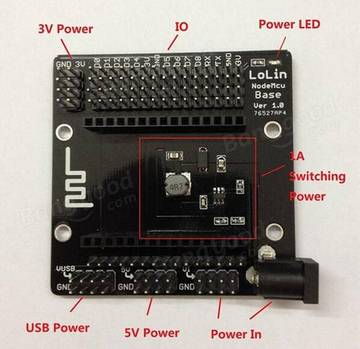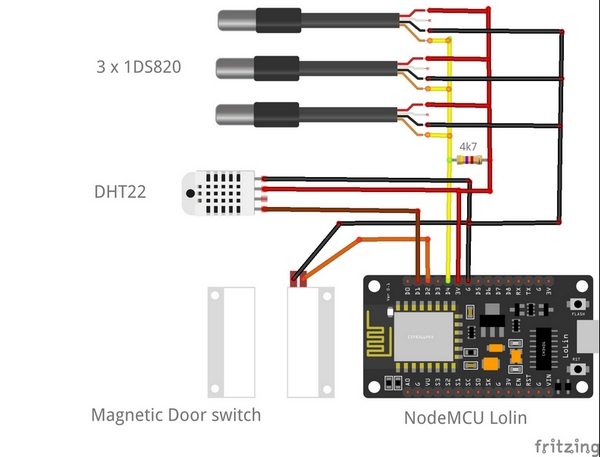B4R Library OneWire - Dallas 1-wire protocol

Discussion in 'B4R Libraries' started by Erel, May 8, 2016.

1. This library is based on the following open source project: http://www.pjrc.com/teensy/td_libs_OneWire.html

It implements Dallas 1-wire protocol.

Example of reading the temperature:
Depends on rOneWire and rRandomAccessFile libraries.
Code:
Sub Process_Globals

Public Serial1 As Serial

Private onewire As OneWire

Private timer1 As Timer

Private bc As ByteConverter

Private type_s As Boolean
End Sub

Private Sub AppStart
Serial1.Initialize(
115200)

Log("AppStart")
onewire.Initialize(
10)
timer1.Initialize(
"timer1_Tick"2000)
timer1.Enabled =
True
End Sub

Sub Timer1_Tick

If onewire.Search(address) = False Then
onewire.ResetSearch

Return

End If

Log("ROM = ", bc.HexFromBytes(address))

Log("CRC is not valid!")

Return

End If

Case 0x10

Log("Chip = DS18S20")
type_s =
True

Case 0x28

Log("Chip = DS18B20")
type_s =
False

Case 0x22

Log("Chip = DS1822")
type_s =
False

Case Else

Log("Device is not a DS18x20 family device.")

Return

End Select
onewire.Reset
onewire.Select(
onewire.Write(
0x44True)

'give it 1 second to read the temperature
End Sub

Private Sub ReadTemparature (u As Byte)
onewire.Reset
onewire.Select(
onewire.Write(
0xBEFalse)

Dim data(12As Byte
9)

Log("Data = ", bc.HexFromBytes(data))

Log("CRC = ", bc.HexFromBytes(Array As Byte(onewire.CRC8(data, 8))))

Dim raw As Int = Bit.Or(Bit.ShiftLeft(data(1), 8), data(0))

If type_s Then
raw =
Bit.ShiftLeft(raw, 3)

If data(7) = 0x10 Then
raw =
Bit.And(raw, 0xFFF0) + 12 - data(6)

End If

Else

Dim cfg As Byte = Bit.And(data(4), 0x60)

If cfg = 0 Then
raw =
Bit.And(raw, Bit.Not(7))

Else if cfg = 0x20 Then
raw =
Bit.And(raw, Bit.Not(3))

Else if cfg = 0x40 Then

Bit.And(raw, Bit.Not(1))

End If

End If

Dim celsius As Double = raw / 16

Dim fahrenheit As Double = celsius * 1.8 + 32

Log("Temperature = "NumberFormat(celsius, 02), " Celsius, " _
,
NumberFormat(fahrenheit, 02), " Fahrenheit")
End Sub
Note that unlike the original C example, the main thread is not blocked here while waiting for the data to be ready.

Attached Files:

• rOneWire.zip
File size:
13 KB
Views:
623
Mostez, GMan, raphaelcno and 2 others like this.
2. Hi,

just to confirm the above code successfully tested. Log:
Code:
AppStart
ROM = 28FF5E1804150334
Chip = DS18B20
Data = 780155003FFF3F10CF051000
CRC = CF
Temperature =
23.50 Celsius, 74.30 Fahrenheit
...

Erel likes this.
3. Confirmed! Works great . Tested in simulation environment though!

Attached Files:

• onewire_example.png
File size:
68.7 KB
Views:
813
jinyistudio likes this.
4. What software did you use for the simulation?

5. Proteus, with arduino library addins

6. Thx

7. work well with arduino...but not work with NodeMCU - ESP8266

With 3 sensor connected on pin D4 (with other pins not work) with 4.7kΩ resistor and voltage 5V directly from NodeMCU(i use nodeMcu Base) i try this ... :
https://www.b4x.com/android/forum/t...18b20-via-inline-c-c-again.76331/#post-486017
... but Temperature is not read correctly:

Code:
ROM = 28FFD97B4A0400B9
Chip = DS18B20
Data = 500555007FFF0C1021000000
CRC =
21
Temperature =
85.00 Celsius, 185.00 Fahrenheit
----------------------------------------------
ROM = 28FF0DFF4E0400B1
Chip = DS18B20
Data = 500555007FFF0C1021000000
CRC =
21
Temperature =
85.00 Celsius, 185.00 Fahrenheit
----------------------------------------------
ROM = 28FFE7FE4C040016
Chip = DS18B20
Data = 500555007FFF0C1021000000
CRC =
21
Temperature =
85.00 Celsius, 185.00 Fahrenheit
----------------------------------------------

Last edited: Dec 4, 2017
8. Can you show a schematic of the connections? Data pin of 1-wire changes quickly from Output to Input and back to output

9. Thanks for the reply. I realized my mistake. I use a NodeMcu BASE like :...and i take voltage 5V directly from NodeMCU Base ... but this is a mistake.
Now i use 3.3V directly from NodeMCu and everything is OK.

Schematic is this:tigrot, Erel and Cableguy like this.
10. Room temperature control?

11. Hope the best success for you pjt! Very nice.

12. This library can only be used for the Arduino of the 8MHz crystal oscillator

13. Dear friends,
I am facing a weird issue. I am using the exact same code given by Erel in first post, using the same circuit as in my post above (#3) , same simulator (proteus) ,but have recompiled the code with B4R 2.51 and not getting to read the sensor! Arduino code in IDE example works OK, meaning the circuit models are fine. Not able to figure out what has changed!14. Tested with B4R v2.51 and Arduino UNO. Works fine here...

15. Many apologies, it must be a model error somehow as the code works perfectly with real hardware, tested with UNO and Wemos D1 R2 both. Nothing to do with B4R release.